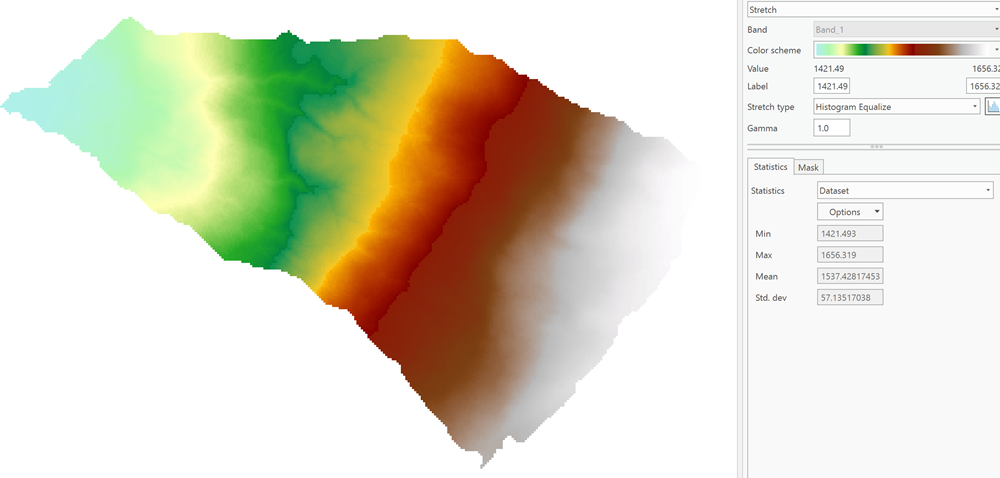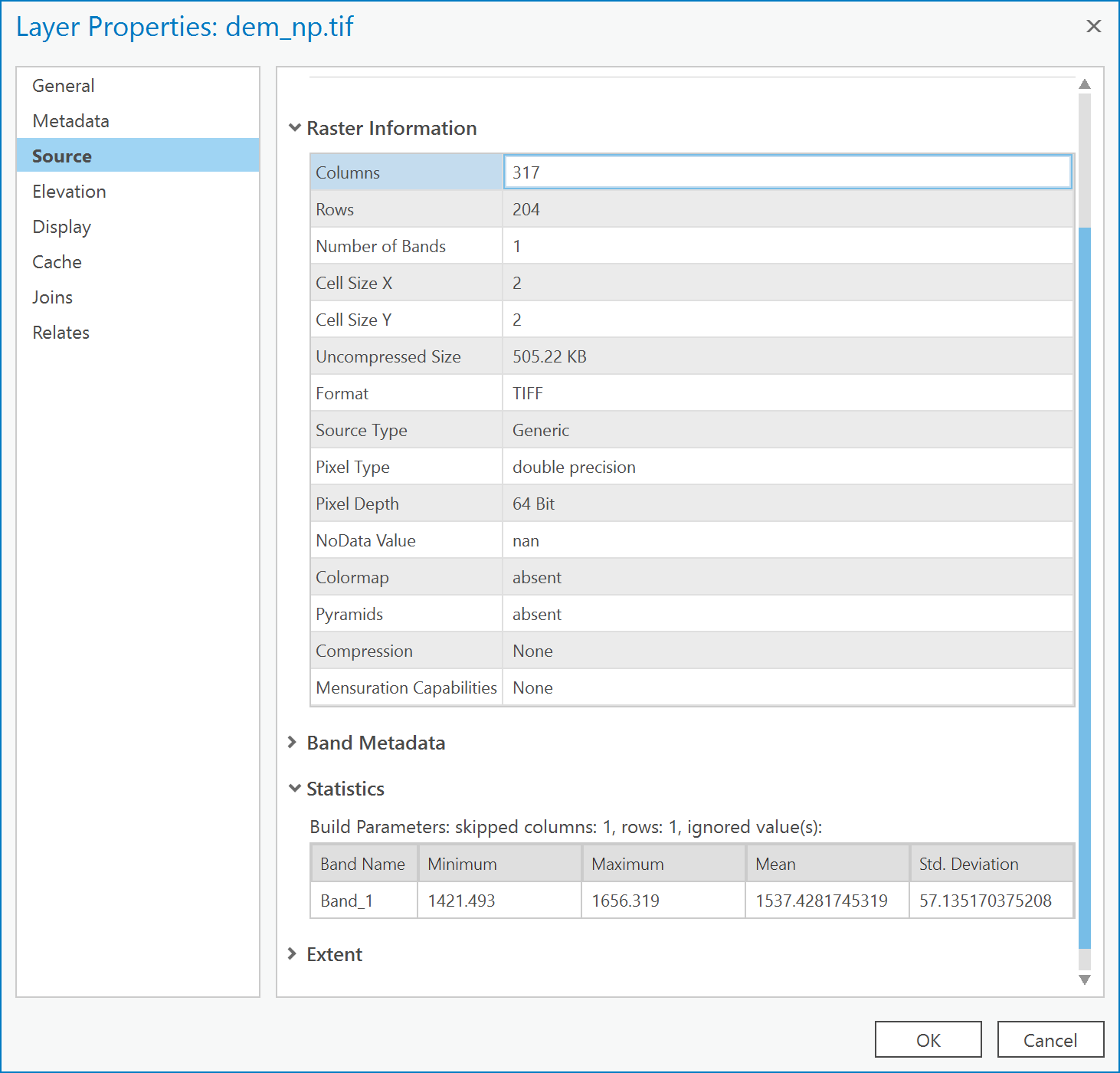685
11
11-19-2018 02:44 PMby
New Contributor

Hi,

I have a DEM in ascii format which I imported into ArcMap using the 'ascii to raster' tool. All values in the ascii file have 3 digits after the decimal point. However, after importing, the raster values do have many more digits (e.g. 1451.623 became 1451.623047...). Does anyone know where the additional digits come from, aka what is happening behind the scenes of the ascii to raster tool?

Thanks!

Marc

11 RepliesbyMVP Esteemed Contributor

Any extra decimals places were already  UNLESS you are confirming that 1451.623 was actually showing in the text file  Otherwise it may be floating point representation but the example you give doesn't show this

for example going from float16 to float64 you can see that the decimal representation is different, but none are gained.

``````z = np.array([1451.623], dtype=np.float16)
print(z)
1452.0

z = np.array([1451.623], dtype=np.float32)
print(z)
1451.623

z = np.array([1451.623], dtype=np.float64)
print(z)
1451.623‍‍‍‍‍‍‍‍‍‍‍``````

So the question might be, what is the final format of your 'raster'…?  an esri grid?  a geodatabase raster?  a *.tif? (recommended).  Do the numbers change with the output format?by
New Contributor

Yes, the value 1451.623 is showing in the text file. The output format doesn't affect the results of the import, i.e. esri grid, tif, geodatabase all result in the same output.

I attached the ascii file, so you might be able to reproduce my observations. Also attached are two screen shots, one from the Identify form (showing the pixel value 1451.623047) and one from the Layer Properties (showing 10 decimal places for the minimum and maximum of the raster).

Thanks again!

MarcbyMVP Esteemed Contributor

I converted your data to a numpy array, it came in as a float32 (32bit floating point values).

I set the array type to float64 and a slice of it looked like this

a[100:300, 100:300]
Out:
array([[1489.567, 1490.179, 1490.441, 1490.702, 1491.003, 1491.728, 1492.366,
1493.299, 1493.946, 1494.314, ..., 1639.082, 1639.969, 1640.586,
1641.29 , 1642.091, 1642.714, 1643.287, 1643.971, 1644.556, 1645.272],
[1490.182, 1490.748, 1491.255, 1491.775, 1492.324, 1492.644, 1493.596,

so I suspect it is just a floating point representation issue. I don't know what they are using behind the scenes.byEsri Regular Contributor

Use IEEE-754 Floating Point Converter  and you'll soon see that 1451.623 is actually 1451.623046875

The ASCIIToRaster tool produces 32 bit rasters.byMVP Esteemed Contributor

Steve are the raster tools 32 bit still?byEsri Regular Contributor

Dan

Yes, however, some of the conversion tools like Point, Polyline and Polygon ToRaster can create a 64 bit (double) raster.  This has been the case for many years, way back in 9.2 or 9.3 I believe. The tool will decide if the output should be 32 or 64 bit. CopyRaster, on the other hand, has a parameter

Esri grid format only supports 32 bit, so you'll rather have to output to fgdb, tif or img.

-StevebyMVP Esteemed Contributor

Steve as you were responding, I used NumPyArrayToRaster… which is my usual route, and things are good

ThanksbyMVP Esteemed ContributorI generated the tiff from your ascii file using numpy as....

``````from arcpy import NumPyArrayToRaster
from arcpy import Point

path = r"C:\Temp\dem.txt"
ncols    =     317
nrows     =    204
xllcorner =    2697732
yllcorner =    1210264
cellsize  =    2
NODATA_value = -9999

a = np.genfromtxt(path, np.float, delimiter=' ', skip_header=6)
a0 = np.where(a==-9999., np.nan, a)

LL = Point(xllcorner, yllcorner)
out = NumPyArrayToRaster(a0, LL, 2.0, 2.0, np.nan)
out.save(r"C:\Temp\dem_np.tif")‍‍‍‍‍‍‍‍‍‍‍‍‍‍‍‍‍‍‍‍‍‍‍‍‍‍‍‍‍‍‍‍‍‍‍‍‍‍‍‍‍‍‍‍‍‍‍‍‍‍‍``````

Statistics yieldSo you can get 64 bit if you need it.byEsri Regular Contributor

you could also skip using ASCIIToRaster and rather use the CopyRaster tool.

Make sure that the ASCII file has a .asc extension and pick PixelType=64 bit